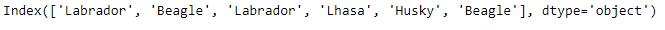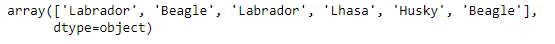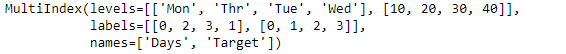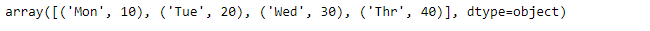# Python | Pandas Index.get_values()

Python is a great language for doing data analysis, primarily because of the fantastic ecosystem of data-centric python packages. Pandas is one of those packages and makes importing and analyzing data much easier.

Pandas` Index.get_values()` function returns the Index data as an numpy.ndarray. It returns one dimensional array for multi-index array.

Syntax: Index.get_values()

Returns : A one-dimensional numpy array of the Index values

Example #1: Use `Index.get_values()` function to return the Index value as a numpy array.

 `# importing pandas as pd ` `import` `pandas as pd ` ` `  `# Creating the Index ` `idx ``=` `pd.Index([``'Labrador'``, ``'Beagle'``, ``'Labrador'``, ` `                     ``'Lhasa'``, ``'Husky'``, ``'Beagle'``]) ` ` `  `# Print the Index ` `idx `

Output :let’s use the `Index.get_values()` function to return the Index data as numpy array.

 `# Returns the labels of Index as numpy array ` `idx.get_values() `

Output :As we can see in the output, the `Index.get_values()` function has returned the index labels as numpy array.

Example #2: Use `Index.get_values()` function on multiindex array.

 `# importing pandas as pd ` `import` `pandas as pd ` ` `  `# Creating the MultiIndex object ` `midx ``=` `pd.MultiIndex.from_arrays([[``'Mon'``, ``'Tue'``, ``'Wed'``, ``'Thr'``], ` `                   ``[``10``, ``20``, ``30``, ``40``]], names ``=``(``'Days'``, ``'Target'``)) ` ` `  `# Print the MultiIndex object ` `midx `

Output :Let’s return the Index labels into one dimensional numpy array format.

 `# Convert the multi-index into one ` `# dimensional numpy array form. ` `midx.get_values() `

Output :As we can see in the output, even the multi-index has been converted into a one-dimensional array form.

My Personal Notes arrow_drop_upCheck out this Author's contributed articles.

If you like GeeksforGeeks and would like to contribute, you can also write an article using contribute.geeksforgeeks.org or mail your article to contribute@geeksforgeeks.org. See your article appearing on the GeeksforGeeks main page and help other Geeks.

Please Improve this article if you find anything incorrect by clicking on the "Improve Article" button below.

Article Tags :

Be the First to upvote.

Please write to us at contribute@geeksforgeeks.org to report any issue with the above content.